## RD Sharma Class 9 Solutions Chapter 19 Surface Areas and Volume of a Circular Cylinder Ex 19.2

These Solutions are part of RD Sharma Class 9 Solutions. Here we have given RD Sharma Class 9 Solutions Chapter 19 Surface Areas and Volume of a Circular Cylinder Ex 19.2

Other Exercises

Question 1.
A soft drink is available in two packs – (i) a tin can with a rectangular base of length 5 cm and width 4 cm, having a height of 15 cm and
(ii) a plastic cylinder with circular base of diameter 7 cm and height 10 cm. Which container has greater capacity and by how much? [NCERT]
Solution:
In first case, in a rectangular container of soft drink, the length of base = 5 cm
and Width = 4 cm
Height = 15 cm
∴ Volume of soft drink = lbh = 5 x 4 x 15 = 300 cm3
and in second case, in a cylindrical container, diameter of base = 7 cm∴ The soft drink in second container is greater and how much greater = 385 cm – 380 cm2 = 85 cm2

Question 2.
The pillars of a temple are cylindrically shaped. If each pillar has a circular base of radius 20 cm and height 10 m. How much concrete mixture would be required to build 14 such pillars? [NCERT]
Solution:
Radius of each pillar (r) = 20 cm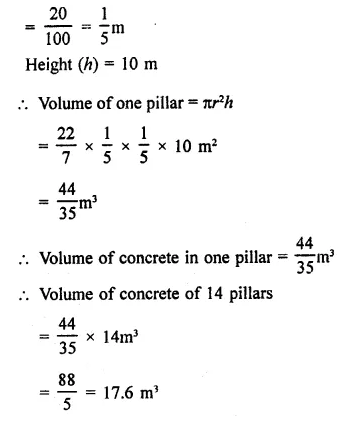Question 3.
The inner diameter of a cylindrical wooden pipe is 24 cm and its outer diameter is 28 cm. The length of the pipe is 35 cm. Find the mass of the pipe, if 1 cm3 of wood has a mass of 0.6 gm. [NCERT]
Solution:
Inner diameter of a cylindrical wooden pipe = 24 cm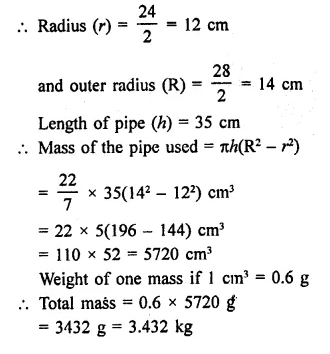Question 4.
If the lateral surface of a cylinder is 94.2 cm2 and its height is 5 cm, find:
(ii) volume of the cylinder [Use π = 3.14] [NCERT]
Solution:
Lateral surface area of a cylinder = 94.2 cm2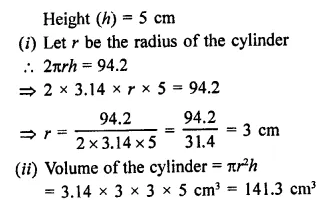Question 5.
The capacity of a closed cylindrical vessel of height 1 m is 15.4 litres. How many square metres of metal sheet would be needed to make it? [NCERT]
Solution:
The capacity of a closed cylindrical vessel = 15.4 l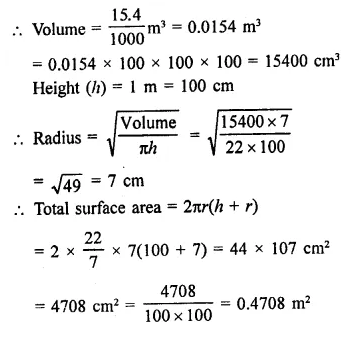Question 6.
A patient in a hospital is given soup daily in a cylindrical bowl of diameter 7 cm. If the bowl is filled with soup to a height of 4 cm, how much soup the hospital has to prepare daily to serve 250 patients? [NCERT]
Solution:
Diameter of the cylindrical bowl = 7 cm
∴ Radius (r) = $$\frac { 7 }{ 2 }$$cm
Level of soup in it = 4 cm
∴ Volume of soup in one bowl for one patient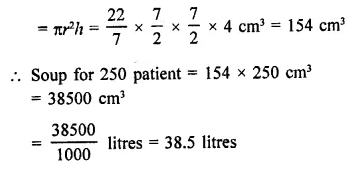Question 7.
A hollow garden roller, 63 cm wide with a girth of 440 cm, is made of 4 cm thick iron. Find the volume of the iron.
Solution:
Width of hollow cylinder (A) = 63 cm
Girth = 440 cm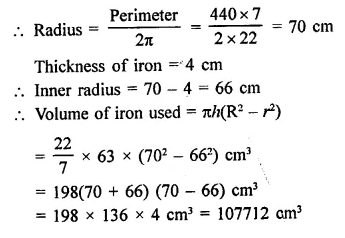Question 8.
The cost of painting the total outside surface of a closed cylindrical oil tank at 50 paise per square decimetre is ₹ 198. The height of the tank is 6 times the radius of the base of the tank. Find the volume corrected to 2 decimal places.
Solution:
Rate of painting = 50 paise per dm2
Total cost = ₹198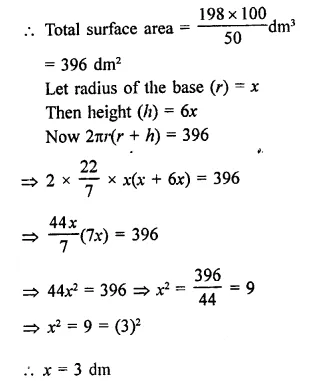Question 9.
The radii of two cylinders are in the ratio 2 : 3 and their heights are in the ratio 5:3. Calculate the ratio of their volumes and the ratio of their curved surfaces.
Solution:
Ratio in radii of two cylinders = 2:3
and ratio in their heights = 5:3
Let radius of the first cylinder (r1) = 2x
and radius of second cylinder (r2) = 3x
and height of first cylinders (h1) = 5y
and height of second cylinder (h2) = 3y
(i) Now volume of the first cylinder = πr2h = π(2x)2 x 5y = 20πx22y
and volume of tlie second cylinder = π(3x)2 x 3y = π x 9×2 x 3y = 27πx2y
Now ratio in their volume
= 20πx2y : 21πx2y = 20 : 27
(ii) Curved surface area of first cylinder = 2πrh = 2π x 2x x 5y =20πxy
and curved surface area of second cylinder = 2π x 3x x = 1 8πxy
∴ Ratio in their curved surface area
= 20πxy : 18πxy = 10 : 9

Question 10.
The ratio between the curved surface area and the total surface area of a right circular cylinder is 1 : 2. Find the volume of the cylinder, if its total surface area is 616 cm2.
Solution:
Ratio in curved surface area and total surface area of a cylinder =1:2
Total surface area = 616 cm2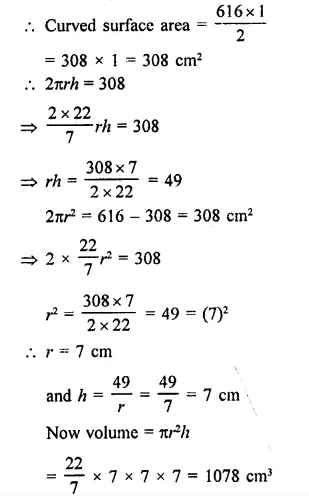Question 11.
The curved surface area of a cylinder is 1320 cm2 and its base had diameter 21 cm. Find the height and the volume of the cylinder. [Use π = 22/7]
Solution:
Curved surface area of a cylinder = 1320 cm2
Diameter of the base = 21 cm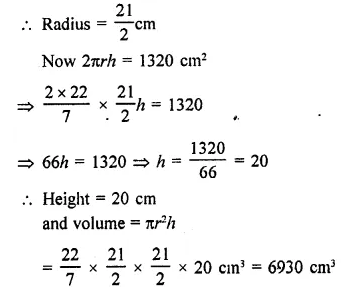Question 12.
The ratio between the radius of the base and the height of a cylinder is 2 : 3. Find the total surface area of the cylinder, if its volume is 1617 cm3.
Solution:
Ratio between radius and height of a cylinder = 2:3
Volume =1617 cm3
Then height (h) = 3x
∴ Volume = πr2h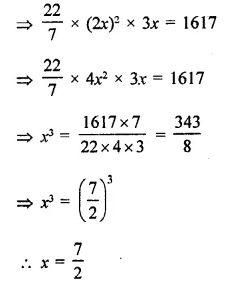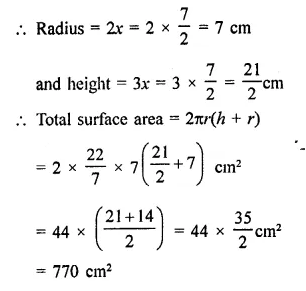Question 13.
A rectangular sheet of paper, 44 cm x 20 cm, is rolled along its length of form a cylinder. Find the volume of the cylinder so formed.
Solution:
Length of sheet = 44 cm
By rolling along length, the height of cylinder (h) = 20cm
and circumference of the base = 44cm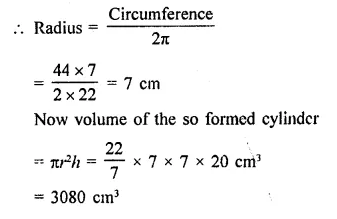Question 14.
The curved surface area of a cylindrical pillar is 264 m2 and its volume is 924 m3. Find the diameter and the height of the pillar.
Solution:
Curved surface area of a pillar = 264 m2
and volume = 924 m3
Let r be the radius and It be height, then 2πrh = 264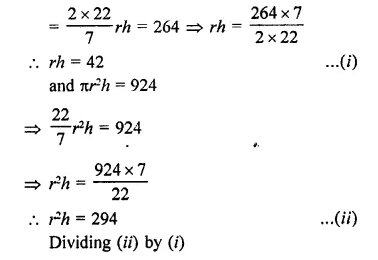Question 15.
Two circular cylinders of equal volumes have their heights in the ratio 1 : 2. Find the ratio of their radii.
Solution:
Volumes of two cylinders are equal Ratio in their height h1 :h2 = 1: 2Question 16.
The height of a right circular cylinder is 10.5 m. Three times the sum of the areas of its two circular faces is twice the” area of the curved surface. Find the volume of the cylinder.
Solution:
Height of a right circular cylinder = 10.5 m
3 x sum of areas of two circular faces
= 2 x area of curved surface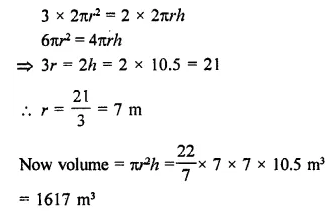Question 17.
How many cubic metres of earth must be dugout to sink a well 21 m deep and 6 m diameter? Find the cost of plastering the inner surface of the well at ₹9.50 per m2.
Solution:
Diameter of a well = 6 m
∴ Radius (r) = $$\frac { 6 }{ 2 }$$ = 3 m
Depth (h) = 21 m
∴ Volume of earth dugout = πr2hQuestion 18.
The trunk of a tree is cylindrical and its circumference is 176 cm. If the length of the trunk is 3 m. Find the volume of the timber that can be obtained from the trunk.
Solution:
Circumference of a cylindrical trunk of a tree = 176 cm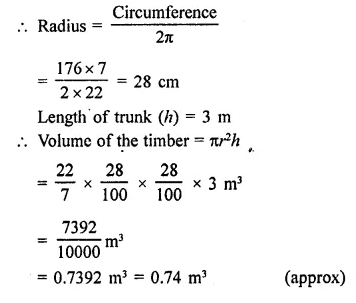Question 19.
A cylindrical container with diameter of base 56 cm contains sufficient water to submerge a rectangular solid of iron with dimensions 32 cm x 22 cm x 14 cm. Find the rise in the level of the water when the solid is completely submerged.
Solution:
Diameter of cylindrical container = 56 cm
∴ Radius (r) = $$\frac { 56 }{ 2 }$$ = 28 cm
Dimensions of a rectangular solid are = 32 cm x 22 cm x 14 cm
∴ Volume of solid = lbh
= 32 x 22 x 14 = 9856 cm3
∴ Volume of water in the container = 9856 cm3
Let h be the level of water, then
πr2h = 9856Question 20.
A cylindrical tube, open at both ends, is made of metal. The internal diameter of the tube is 10.4 cm and its length is 25 cm. The thickness of the metal is 8 mm everywhere. Calculate the volume of the metal.
Solution:
Length of metallic tube = 25 cm
Inner diameter = 10.4 cm
∴ Radius (r) = $$\frac { 10.4 }{ 2 }$$ = 5.2 cm
Thickness of metal = 8 mm
∴ Outer radius (R) = 5.2 + 0.8 = 6.0 cm
Volume of metal used = π(R2 – r2) x h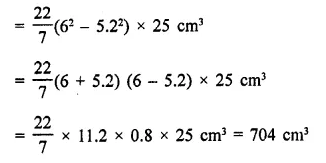Question 21.
From a tap of inner radius 0.75 cm, water flows at the rate of 7 m per second. Find the volume in litres of water delivered by the pipe in one hour.
Solution:
Inner radius of a tap = 0.75 cm
Speed of flow of water in it = 7 m/s
Time = 1 hour
∴ Length of flow of water (h)
= 7 x 60 x 60 m = 25200 m
∴ Volume of water = πr2h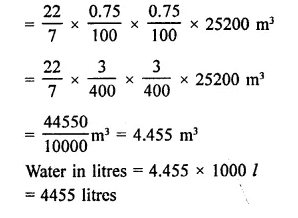Question 22.
A rectangular sheet of paper 30 cm x 18 cm can be transformed into the curved surface of a right circular cylinder in two ways i.e., either by rolling the paper along its length or by rolling it along its breadth. Find the ratio of the volumes of the two cylinders thus formed.
Solution:
Size of rectangular sheet = 30 cm x 18 cm
∴ Length of sheet = 30 cm
By folding length wise,
Height = 18 cm
and circumference = 30 cm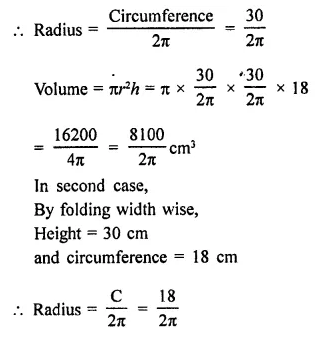Question 23.
How many litres of water flow out of a pipe having an area of cross-section of 5 cm2 in one minute, if the speed of water in the pipe is 30 cm/sec?
Solution:
Area of the cross-section of the pipe = 5 cm2
Speed of water flow = 30 cm/sec
Period = 1 minute
∴ Flow of water in 1 minute = 30 x 60 cm = 1800 cm
Area of mouth of pipe = 5 cm2
∴ Volume = 1800 x 5 = 9000 cm3
Volume of water in litres = 9000 ml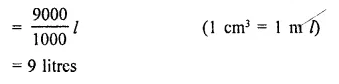Question 24.
Find the cost of sinking a tubewell 280 m deep, having diameter 3 m at the rate of ₹3.60 per cubic metre. Find also the cost of cementing its inner curved surface at ₹2.50 per square metre.
Solution:
Depth of well (h) = 280 m
Diameter = 3 m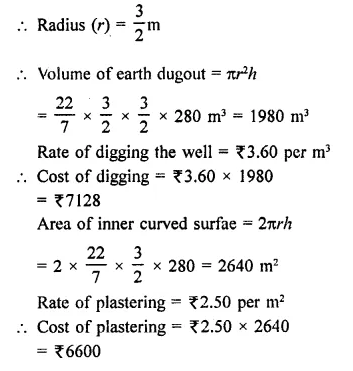Question 25.
Find the length of 13.2 kg of copper wire of diameter 4 mm, when 1 cubic cm of copper weighs 8.4 gm.
Solution:
Weights of copper wire = 13.2 kg
Diamter = 4 mmQuestion 26.
A solid cylinder has a total surface area of 231 cm2. Its curved surface area is $$\frac { 2 }{ 3 }$$ of the total surface area. Find the volume of the cylinder.
Solution:
Surface area of solid cylinder = 231 cm2
and curved surface area = $$\frac { 2 }{ 3 }$$ of 231 cm2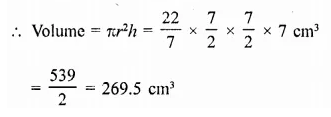Question 27.
A well with 14 m diameter is dug 8 m deep. The earth taken out of it has been evenly spread all around it to a width of 21 m to form an embankment. Find the height of the embankment.
Solution:
Diameter of a well = 14 m
∴ Radius (r) = y = 7 m
Depth (h) = 8 m
∴ Volume of the earth dugout = πr2h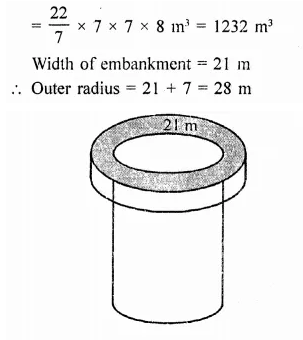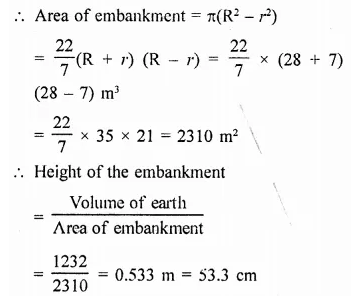Question 28.
The difference between inside and outside surfaces of a cylindrical tube 14 cm long is 88 sq. cm. If the volume of the tube is 176 cubic cm, find the inner and outer radii of the tube.
Solution:
Length of cylindrical tube = 14 cm
Difference betveen the outer surface and inner surface = 88 cm2
and volume of the tube = 176 cm3
Let R and r be the outer and inner radius of the tube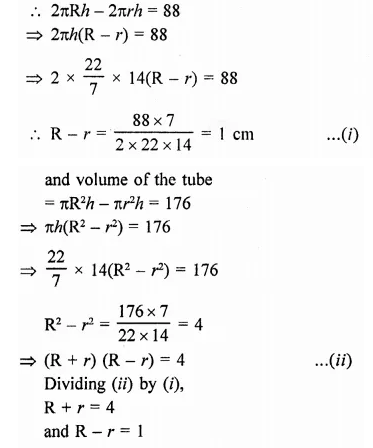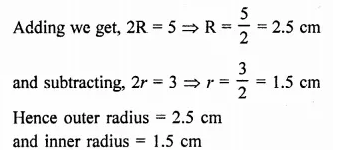Question 29.
Water flows out through a circular pipe whose internal diameter is 2 cm, at the rate of 6 metres per second into a cylindrical tank. The radius of whose base is 60 cm. Find the rise in the level of water in 30 minutes?
Solution:
Internal diameter of the pipe = 2 cm
∴ Radius (r) = $$\frac { 2 }{ 2 }$$ = 1 cm
Speed of water flow = 6m per second Water in 30 minutes (h) = 6 x 60 x 30 m = 10800 m
Volume of water = πr2h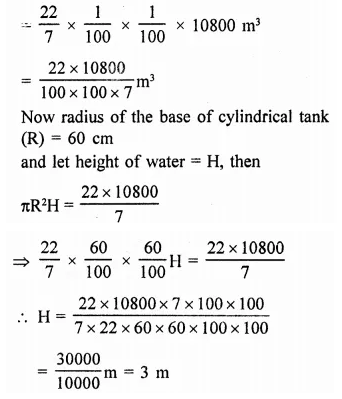Question 30.
A cylindrical water tank of diameter 1.4 m and height 2.1 m is being fed by a pipe of diameter 3.5 cm through which water flows at the rate of 2 metre per second. In how much time the tank will be filled?
Solution:
Diameter of cylindrical tank = 1.4 m
∴ Radius (r) = $$\frac { 1.4 }{ 2 }$$ = 0.7 m
and height (h) = 2.1 m
∴ Volume of water in the tank = πr2h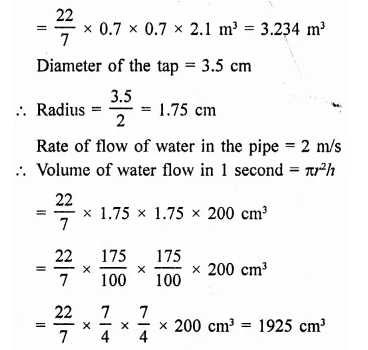Question 31.
The sum of the radius of the base and height of a solid cylinder is 37 m. If the total surface area of the solid cylinder is 1628 m2. Find the volume of the cylinder.
Solution:
Sum of radius and height of a cylinder = 37 m
Let r be the radius and h be the height, then r + h = 37m …(i)
Total surface area of a solid cylinder = 1628m3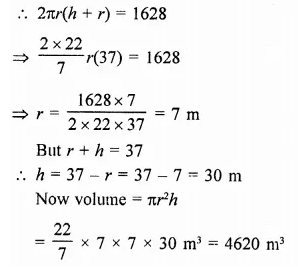Question 32.
A well with 10 m inside diameter is dug 8.4 m deep. Earth taken out of it is spread all around it to a width of 7.5 m to form an embankment. Find the height of the embankment.
Solution:
Diameter of the well = 10 m 10
∴ Radius (r) = $$\frac { 10 }{ 2 }$$ = 5 m
Depth (h) = 8.4 m
∴ Volume of earth dugout = πr2hHope given RD Sharma Class 9 Solutions Chapter 19 Surface Areas and Volume of a Circular Cylinder Ex 19.2 are helpful to complete your math homework.

If you have any doubts, please comment below. Learn Insta try to provide online math tutoring for you.